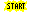´

Schubert polynomials

In Symmetrica there are many routines to do computations with Schubert polynomials. These are generalizations of Schur polynomials. In Symmetrica there are routines available to do the following tasks:compute Schubert polynomialsproduct of Schubert polynomialscompute double Schubert polynomialscompute non commutative Schubert polynomials....